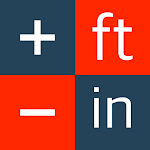Package: com.knownpoint.app.feetinchplus

• Convert measurements in inches, feet, yards, meters, miles, kilometers, acres, square feet, hectare and more
• Enter and calculate feet-inches and inches as fractions
• Option to round off inches to 1/32nd, 1/16th, 1/8th, etc.
• Add and subtract measurements in different units
• Complete Fraction Calculator: add, subtract, multiply, and divide fractions
• One-click to convert between decimal and fraction
• Access trigonometry and other advanced functions with swipes
• History Tape saves past calculations with easy recall function and starred memory function
• Backspace and clear functions

Everyday uses include:

• Length calculation in feet and inches such as 10 ft minus 3 ½ in
• Finding out the size of a room or a deck by multiplying length by width, example, 42 ½ ft by 112 ¾ ft
• Get the remainder when dividing, 100 yards remainder 3 yards.
• Helping your kids with fraction math including converting decimal to fraction and back

Technical Support

We will be happy to address feedback regarding product features, technical support, defects, and enhancement requests.